Goseeko blog# What is the convergence of sequence and series?

## Overview (sequence and series)

A function f : N  , where S is a non-empty set , is called sequence , for each nϵN.

We write sequence as f(1) , f(2) , f(3) , f(4)……….f(n).

Any sequence f(n) can be denoted as <f(n)> or {f(n)} or (f(n)).

Suppose f(n) = sn

Then it can be written as –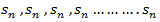and can be denoted as <sn >or  {sn } or ( sn)

sn is the n’th term of the sequence.

Example:  suppose we have a sequence – 1 , 4 , 9 , 16 ,……….. and its n’th term is n2.

The sequence, we can write as  <n2 >

## Types of sequences

1. Finite sequence- A sequence which has finite number of terms is finite sequence.

2. Infinite sequence-  A sequence which is not finite , called infinite sequence.

Limit of a Sequence– A sequence <sn> is said to tend to limit “l” , when given any positive number ‘ϵ’ , however small , we can always find a integer ‘m’ such that |sn – l| <ϵ , for every for all, n≥m , and we can define this as follows,

Example: If    , then the limit of  will be-

Hence the limit of the sequence is 1/2.

## Some important limits to remember for sequence and series

Convergent sequence- A sequence Sn is convergent when it tends to a finite limit. That means the limit of a sequence Sn will be always finite in case of convergent sequence.

Divergent sequence- when a sequence tends to ±∞ then it is divergent sequence.

Oscillatory sequence-  when a sequence neither converges nor diverges then it is an oscillatory sequence.

Note- a sequence which neither converges nor diverges , is called oscillatory sequence.

A sequence is null, when it converges to zero.

Example-1:  consider a sequence 2, 3/2 , 4/3 , 5/4, …….. here Sn =  1 + 1/n

Sol. As we can see that the sequence Sn is convergent and has limit 1.

According to def.

Example-2: consider a sequence Sn=  n² + (-1).

Sol. Here we can see that, the sequence Sn is divergent  as it has infinite limit.

## Series

Infinite series- If   is a sequence , then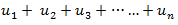is called the infinite series.

We denote this by

Examples of infinite series-

Covergent series –  suppose n→∞ , Sn→ a finite limit  ‘s’  , then the series Sn is said to be convergent .

We can denote it as,

Divergent series–  when Sn tends to infinity then the series is said to be divergent.

Oscillatory series-  when Sn does not tends to a unique limit (finite or infinite) , then it is called Oscillatory series.

## Properties of infinite series

1. the convergence and divergence of an infinite series is unchanged addition od deletion of a finite number of terms from it.

2. if positive terms of convergent series change their sign , then the series will be convergent.

3. Let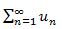converges to s , let k be a non-zero fixed number  then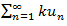converges to ks.

4. Letconverges to ‘l’ andconverges to ‘m’.

Example-1: check whether the series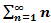is convergent or divergent. Find its value in case of convergent.

Sol.  As we know that,

Sn =Therefore,

Sn =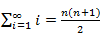Now find out the limit of the sequence,

Here the value of the limit is infinity, so that the series is divergent as sequence diverges.

Example-2: check whether the series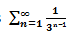is convergent or divergent. Find its value in case of convergent.

Sol. The general formula for this series is,

We get

Hence the series is convergent and its values is 3/2.

Example: Test the convergence of the series-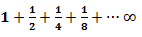Sol. Here we can see that the given series is in geometric progression

As its first term is 1 and common ratio is ½.

Then we know that the sum of n terms of a geometric progression is-

Hence the limit will be-

So that the series is convergent.

## General properties of series

The general properties of series are-

1. The nature of a series does not change by multiplication of all terms by a constant k.

2. The nature of a series does not change by adding or deleting of a finite number of terms.

Interested in learning about similar topics? Here are a few hand-picked blogs for you!